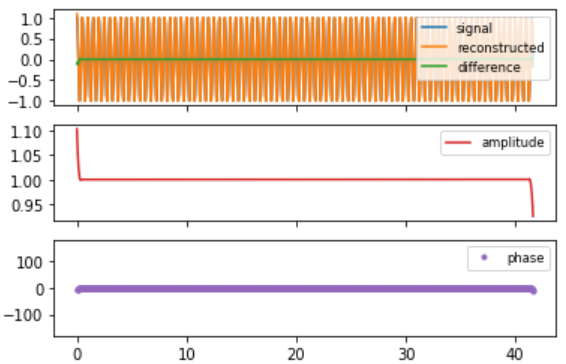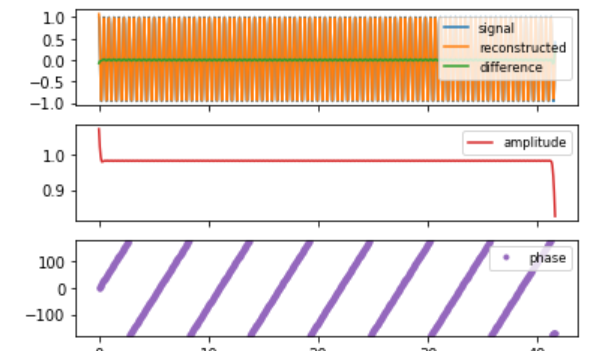# Complex Demodulation Phase and Amplitude Plot

• Last Updated : 14 Aug, 2021

### Complex Demodulation Phase Plot

In the frequency analysis of time series models, a common model is a sinusoidal wave:Attention reader! Don’t stop learning now. Get hold of all the important Machine Learning Concepts with the Machine Learning Foundation Course at a student-friendly price and become industry ready.

where, ∝ is the amplitude, phi is the phase shift and omega is the dominant frequency. The goal of the complex demodulation plot is to improve the frequency estimate.

The complex demodulation plots formed by two components:

• Vertical axis: Phase
• Horizontal axis: Time

#### Importance of a Good Initial Estimate for the Frequency:

The non-linear fitting for the sinusoidal wavesThe above equation is sensitive to good initial values. The initial value of frequency omega can be obtained by the spectral plot. The complex demodulation phase plot is used to assess whether this estimate is adequate. If the estimate is not adequate then whether it should be increased and decreased.

### Complex Demodulation Amplitude Plot:

In the frequency analysis of time series models, a common model is a sinusoidal wave:where, ∝ is the amplitude, phi is the phase shift and omega is the dominant frequency.

If the slope of the complex demodulation amplitude plot is not zero, then the above equation is finally replaced by the model.where, ai is some type of linear model fit with standard least squares. The most common case is linear fit, that is the model becomes as follows:The complex demodulation amplitude plot is formed by

• Vertical axis: Amplitude
• Horizontal axis: Time

The complex amplitude demodulation plot answers the following questions:

• Does amplitude change over time?
• Is there any start-up effect that led to the amplitude being unstable at the start?
• Is there any outliers in amplitude?

## python3

 # codeimport numpy as npimport matplotlib.pyplot as plt def gen_test_data(periods, noise=0, rotary=False, npts=1000, dt=1.0/24):    """    Generate a simple time series for testing complex demodulation.         *periods* is a sequence with the periods of one or more        harmonics that will be added to make the test signal.        They can be positive or negative.    *noise* is the amplitude of independent Gaussian noise.       *rotary* is Boolean; if True, the test signal is complex.    *npts* is the length of the series.    *dt* is the time interval (default is 1.0/24)         Returns t, x: ndarrays with the test times and test data values..    """                 t = np.arange(npts, dtype=float) * dt         if rotary:        x = noise * (np.random.randn(npts) + 1j * np.random.randn(npts))    else:        x = noise * np.random.randn(npts)     for p in periods:        if rotary:            x += np.exp(2j * np.pi * t / p)        else:            x += np.cos(2 * np.pi * t / p)         return t, x   def complex_demodulation(t, x, central_period, hwidth = 2):    """    Complex demodulation of a real or complex series, *x*    of samples at times *t*, assumed to be uniformly spaced.         *central_period* is the period of the central frequency        for the demodulation.  It should be positive for real        signals. For complex signals, a positive value will        return the CCW rotary component, and a negative value        will return the CW component (negative frequency).        Period is in the same time units as are used for *t*.     *hwidth* is the Blackman filter half-width in units of the        *central_period*.  For example, the default value of 2        makes the Blackman half-width equal to twice the        central period.         Returns a dictionary.    """             rotary = x.dtype.kind == 'c'  # complex input         # Make the complex exponential for demodulation:    c = np.exp(-1j * 2 * np.pi * t / central_period)         product = x * c         # filter half-width number of points    dt = t - t    hwpts = int(round(hwidth * abs(central_period) / dt))    nf = hwpts * 2 + 1    x1 = np.linspace(-1, 1, nf, endpoint=True)    x1 = x1[1:-1]   # chop off the useless endpoints with zero weight    w1 = 0.42 + 0.5 * np.cos(x1 * np.pi) + 0.08 * np.cos(x1 * 2 * np.pi)    ytop = np.convolve(product, w1, mode='same')    ybot = np.convolve(np.ones_like(product), w1, mode='same')    demod = ytop/ybot    if not rotary:           # The factor of 2 below comes from fact that the        # mean value of a squared unit sinusoid is 0.5.        demod *= 2         reconstructed = (demod * np.conj(c))    if not rotary:        reconstructed = reconstructed.real             if np.sign(central_period) < 0:        demod = np.conj(demod)        # This is to make the phase increase in time        # for both positive and negative demod frequency        # when the frequency of the signal exceeds the        # frequency of the demodulation.         d = {'t':t,'signal' : x,'hwpts' : hwpts,'demod': demod,'reconstructed' : reconstructed}    return d   def plot_demodulation(dm):    fig, axs = plt.subplots(3, sharex=True)    resid = dm.get('signal') - dm.get('reconstructed')    if dm.get('signal').dtype.kind == 'c':        axs.plot(dm.get('t'), dm.get('signal').real, label='signal.real')        axs.plot(dm.get('t'), dm.get('signal').imag, label='signal.imag')        axs.plot(dm.get('t'), resid.real, label='difference real')        axs.plot(dm.get('t'), resid.imag, label='difference imag')    else:           axs.plot(dm.get('t'), dm.get('signal'), label='signal')        axs.plot(dm.get('t'), dm.get('reconstructed'), label='reconstructed')        axs.plot(dm.get('t'), dm.get('signal') - dm.get('reconstructed'), label='difference')         axs.legend(loc='upper right', fontsize='small')         axs.plot(dm.get('t'), np.abs(dm.get('demod')), label='amplitude', color='C3')    axs.legend(loc='upper right', fontsize='small')         axs.plot(dm.get('t'), np.angle(dm.get('demod'), deg=True), '.', label='phase',                color='C4')    axs.set_ylim(-180, 180)    axs.legend(loc='upper right', fontsize='small')         for ax in axs:        ax.locator_params(axis='y', nbins=5)    return fig, axs def test_demodulation(periods, central_period,                 noise=0,                 rotary=False,                 hwidth = 1,                 npts=1000,                 dt=1.0/24):         t, x = gen_test_data(periods, noise=noise, rotary=rotary,                     npts=npts, dt=dt)    dm = complex_demodulation(t, x, central_period, hwidth=hwidth)    fig, axs = plot_demodulation(dm)    return fig, axs, dm# Example 1test_demodulation([12.0/24], 12.0/24);# Example 2test_demodulation([11.0/24], 12.0/24)Example 1: Signal, amplitude demodulation, and phase demodulationExample 2: Signal, amplitude demodulation, and phase demodulation

My Personal Notes arrow_drop_up